# Calculation of Solid Oral Doses and Dosages

Filed under Basic Science

rate 1 star rate 2 star rate 3 star rate 4 star rate 5 starChapter 7

# Calculation of Solid Oral Doses and Dosages

Pretest

## Calculating Solid Oral Medication Doses

To provide the necessary medication for the administration of a solid oral dose, the label must be read carefully and the correct medication must be used in dispensing the drug. After choosing the appropriate medication, the pharmacy technician must be sure to calculate the amount of medicine to be given to the patient both in the individual dose and the entire amount of medication, or dosage. Care is necessary to supply the treatment prescribed for patient safety. The calculation of a dose depends on the amount of medicine that has been ordered at a given time, whereas dosage indicates the total amount of medicine that is necessary for the complete order or prescription. This chapter covers calculating both doses and dosages. Just as a reminder, the label on the medication provided and the prescribed medication must be either in the same measurement system or a form that can be converted to provide the prescribed medicine dose and dosage. Another important rule of thumb is that when converting among measurement systems, be sure the final conversion is in the same measurement system as that found on the label of the medication. In other words, if you are converting between milligrams and grams and the label on the medication is in grams per tablet, the final answer should be in grams per tablet or the number of tablets. Finally, be sure that the medication names are exactly the same, because many medications have sound-alike or look-alike names that are similar. If there is ever a doubt about the medicine to be used or if the calculation does not seem to be what you expected it to be, obtain clarification from the pharmacist before preparing the medicine, a practice that is always safe, important, and acceptable. The pharmacist—the person ultimately responsible for dispensing the medication—would prefer to answer questions before medication preparation rather than make a medication error by dispensing incorrect doses or medications.

More rules that should be considered when calculating medication dosage follow:

Drug calculations may be obtained by using one of three methods. The method that you feel the most comfortable using is the correct method for you to use. One method is using ratio and proportion (discussed in Chapter 2). Dimensional analysis is an extended form of ratio and proportion and may also be used for calculating a dose of medication. Finally, the following formula may be used for calculations:

< ?xml:namespace prefix = "mml" />DD(Dose desired)DH(Dose on hand)×Qty(Quantity or form)=Dose to be given(DG)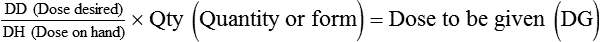In the next section, each problem will be calculated using each of the three methods. Again, find the method with which you feel the most comfortable and use it for all your calculations. Always using the same methodology for calculations will assist in avoiding possible confusion during calculations.

### Calculating Medications Using Ratio and Proportion

When using the proportional method of calculating doses, the dosage strength available (DA) and the dosage form (DF) must be one ratio, and the dose ordered (DO) and the dose to be given (DG) must be the second ratio in the proportion. Remember that in using a mathematical equation for ratio and proportion, the ratio shows the relationship between two numbers, and proportion shows the relationship between two ratios. When setting up a problem for calculation by ratio and proportion, the formula then appears as follows:

DA(Dosage available):DF(Dosage form)::DO(Dose ordered):DG(Dose to be given)Remember that these relationships may also be written as fractional units if this is easier for your calculations. A fractional formula is as follows: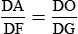Also remember that when part of the ratio is missing, the unknown number is represented by “x”. If a calculation is necessary and one of the components of the proportion is missing, the missing part or x can be found by filling in the known parts of the proportion and then completing the problem as with any proportional problem. To use proportion, the dosage available (DA) and the dose ordered (DO) must be in the same measurement system. If the systems differ, conversion must be done to bring these components into the same system. (See Chapter 4 for help in remembering the measurement systems and Chapter 5 for help in converting among systems.) Later in this chapter you will calculate problems requiring conversion. Now you can begin performing calculations with medications in the same system.

Example 7-1

In this proportion, if you know that 250 mg (DA) of a medication is found in 1 capsule (DF) and the order reads for the patient to be given 500 mg (DO), the ratio would look like this:

250mg(DA):1cap(DF)::500mg(DO):x(DG)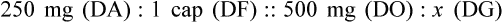OR

250mg(DA)1cap(DF)=500mg(DO)x(DG)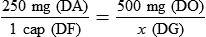Now multiply the means versus extremes or cross-multiply if in fractional components.

Drop the “mg” because this appears in both parts of the equation, and the equation now looks like this:

250x=500×1cap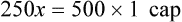x=500cap250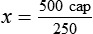x = 2 cap, or the prescribed amount of medication to be given is 2 capsules

Let’s try another medication order as an example, but this time we will use measurements in the apothecary system.

Example 7-2

gr14(DA):1tab(DF)::gr34(DO):x(DG)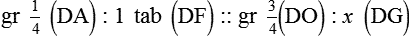OR

gr1/4(DA)1tab(DF)=gr3/4(DO)x(DG)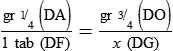As previously, drop the “gr” because this appears on both sides of the equation. Again, either multiply means and extremes or cross-multiply the fractional components. The resultant formula is as follows:

14x=34×1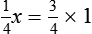x=34×41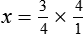x=31or3tablets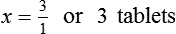### Calculating Medications Using Dimensional Analysis

If the previous problems were calculated in dimensional analysis, the first problem would read as follows:

Example 7-3

xcaps=1cap250mg×500mg1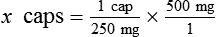xcaps=1×21×1orxcaps=21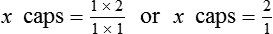xcaps=2capsules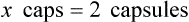Example 7-4

xtabs=1tabgr1/4=gr3/41Dose to be given will be3tablets.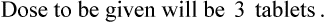### Calculating Medications Using the Formula Method

Many professionals use the formula method to calculate doses. Using the formula, replace each component of the formula with the correct information and then calculate the problem. This is the same means of replacement as is used with ratio and proportion, but the formula is used for placing the given amounts. The formula follows:

DD(Dose desired)DH(Dose on hand[DA])×Qty(Quantity[DF])=Dose to be given(DG)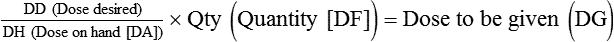Quantity in solid medications will appear as capsules or tablets, whereas in liquid form the quantity may be found in the number of mg, g, or other weight measurement in the volume of medication, or may be given mL, drops, or other liquid volume measurements.

Example 7-5

500mg(DD)250mg(DA)×1cap(Qty[DF])=Dose to be given(DG)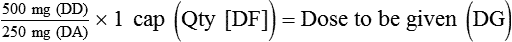500250×1cap=DG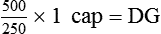500÷250=2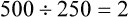2×1=2capsules to be given